# Java Program to Find Sum of Digits Until Single Digit

In this tutorial you will learn about the Java Program to Find Sum of Digits Until Single Digit and its application with practical example.

In this tutorial, we will learn to create a Java Program to Find sum of digits Until Single Digit in a number using Java programming.

## Prerequisites

Before starting with this tutorial we assume that you are best aware of the following Java programming topics:

• Java Operators.
• Basic Input and Output function in Java.
• Class and Object in Java.
• Basic Java programming.
• If-else statements in Java.
• For loop in Java.
• Inbuilt functions and user define functions.

## Sum of Digits of a number until single Digit.

Here we will find the sum of digits until the number becomes single digit.
Example:-Suppose we have taken a number = 346.
The sum of digits of 346 = 3+4+6 = 13.
Now the number 13 is of two digits number so we need to do again we will find the sum of digits of the number,
The sum of digits of 13 = 1+3 = 4
Finally 4 is single-digit so sum of the number until single digit of 346 is 4.

## Java Program to Find Sum of Digits Until Single Digit

In this program we will learn to create a program that will find sum of digits until single digit of a given number. Firstly we declare required variable and also initiates required values in variable. Next we take value from user at run time and then after we will find the sum of all digit until single digit sum.

## Output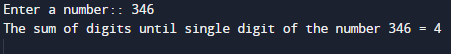In the above program, we have first declared and initialized a set variables required in the program.

• number= it will hold entered number.
• firstdigit= it will hold single digit sum.
• sum= it will hold sum of digit.
• enddigit= it will hold find last digit.
• total=it will add the  values.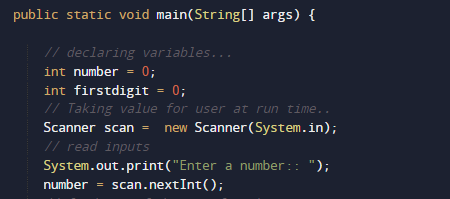Here we first declared and initialized set of variables required in the program. Then we will take a value from user at run time.After that pass the given number to function named Sumation(number).where we going to calculate sum of all digit of a given number.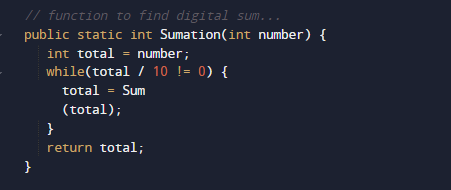within the function we pass total to function Sum()  where we calculate sum of digit to unit place.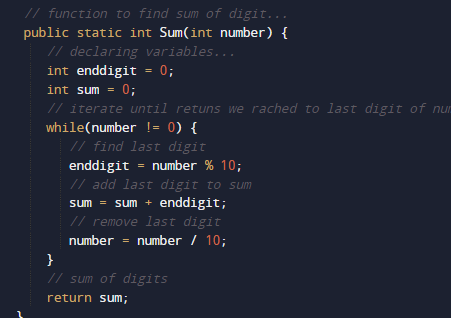this function will return the digit sum to main function where we print the result.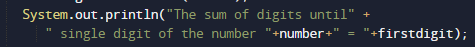In this tutorial we have learn about the Java Program to Find Sum of Digits Until Single Digit and its application with practical example. I hope you will like this tutorial.